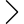80 products found
• s
• m
• l
• xl
• 2xl
• s
• m
• l
• xl
• s
• m
• l
• xl
• 2xl
• s
• m
• l
• xl
• 2xl
• s
• m
• l
• xl
• 2xl
• s
• m
• l
• xl
• 2xl
• s
• m
• l
• xl
• 2xl
• s
• m
• l
• xl
• 2xl
EXPOSED SEAMS PANEL T-SHIRT
\$179.00 \$125.30
• s
• m
• l
• xl
• 2xl
\$189.00 \$132.30
• s
• m
• l
• xl
• s
• m
• l
• xl
• 2xl
• s
• m
• l
• xl
• s
• m
• l
• xl
• s
• m
• l
• xl
MANTA CARGO TROUSERS
\$299.00 \$209.30
• s
• m
• l
• xl
• 2xl
EDEN PRINTED PULLOVER
\$249.00 \$174.30
• s
• m
• l
• xl
• s
• m
• l
• xl
EXPOSED SEAMS PANEL T-SHIRT
\$209.00 \$146.30
• s
• m
• l
• xl
• s
• m
• l
• xl
MANTA CARGO TROUSERS
\$299.00 \$209.30
• s
• m
• l
• xl
• s
• m
• l
• xl
• s
• m
• l
• xl
• 2xl
EXPOSED SEAMS PANEL T-SHIRT
\$179.00 \$125.30
• s
• m
• l
• xl
• s
• m
• l
• xl
• s
• m
• l
• xl
• s
• m
• l
• xl
• s
• m
• l
• xl
• s
• m
• l
• xl
• s
• m
• l
• xl
CONTRAST PANEL LOUNGE PANTS
\$269.00 \$188.30
• s
• m
• l
• xl
• s
• m
• l
• xl
CONTRAST PANEL LOUNGE PANTS
\$269.00 \$188.30
• s
• m
• l
• xl
LAYERED PANEL CARGO PANTS
\$319.00 \$223.30
• s
• m
• l
• xl
• s
• m
• l
• xl
• s
• m
• l
• xl
• s
• m
• l
• xl
• s
• m
• l
• xl
• s
• m
• l
• xl
• 2xl
• 1
• 2
•